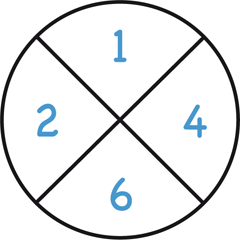24

Players: Two or more

The players choose four numbers from 1 to 10, and then compete to combine the numbers with operators to make 24.

Description

Two players each secretly write two numbers between 1 and 10 on a piece of paper. They then simultaneously reveal the numbers, and try to reach exactly 24 by combining the numbers using only addition, subtraction, multiplication, division, and brackets.

The first player to announce a solution wins.

Example

Traditionally the numbers are written in a circle; for example, a possible set of numbers is:All the numbers must be used, so this cannot simply be solved with (4 * 6). One possible solution is: 4 * 6 * (2 - 1).

Also, division is allowed to produce a non-integer result (unlike in Number Countdown), so these numbers could also be solved as: 4 / ((2 - 1) / 6).

Other topics

History

Strategy

Other games like 24: Number Countdown